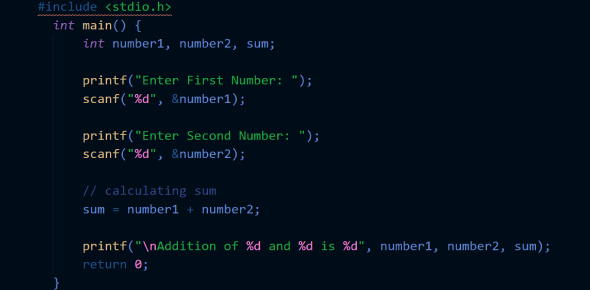C Programming Questions: Trivia Quiz! Exam

40 Questions | Total Attempts: 809SettingsDo you think you are an expert with C Programming Language? This quiz highlights specific pertinent questions such as the maximum value of an integer constant when C Language was created, what is permitted in C arithmetic instruction, and a collection of data items called elements. C language is basic, yet it can be challenging to learn. This quiz is the ultimate test on C Programming.

• 1.
The Expression, A=30*1000+2768 evaluates to ________________.
• A.

-32768

• B.

32768

• 2.
The maximum value that an integer constant can have is ____________________.
• A.

-3276700%

• B.

3276700%

• 3.
C Language came into existence in the year ____________________.
• A.

1971

• B.

1972

• 4.
Macros are defined using ___________________ statement.
• A.

#define

• B.

#include

• 5.
____________________ of the following is allowed in a C arithmetic instruction.
• A.

()

• B.

[]

• 6.
The Maximum width of a C variable name can be ________________.
• A.

20 Character

• B.

32 Character

• 7.
________________ are the ASCII values for number 0-9.
• A.

23-45

• B.

48-57

• 8.
In the C Language ‘A’ represents ________________.
• A.

A Digit

• B.

A Character

• 9.
To find factorial of A by using while loop,  A will be ________________.
• A.

A

• B.

A>0

• 10.
The goto statement causes control to go to ___________________.
• A.

An operator

• B.

A label

• 11.
The Symbol && is read as ____________________.
• A.

AND

• B.

OR

• 12.
An ___________________ is a collection of data items its called elements.
• A.

Array

• B.

Structure

• 13.
___________________ does not represent a valid storage class in “C”.
• A.

Automatic

• B.

Union

• 14.
In ‘C’ logical AND operator is represented as ___________________.
• A.

Binary

• B.

DOS

• 15.
___________________ keyword is used to exit loops at arbitrary spots.
• A.

Break

• B.

CLOSE

• 16.
The ___________________ loop executes at least once.
• A.

Break

• B.

Stop

• 17.
In face “++” has a higher precendence than “*”.
• A.

True

• B.

False

• 18.
Null will be defined as something else.
• A.

True

• B.

False

• 19.
Scanf function is used to display information on the Monitor.
• A.

True

• B.

False

• 20.
A for loop is used to execute a section of code a specific number of times.
• A.

True

• B.

False

• 21.
A do while loop is very similar to a while loop.
• A.

True

• B.

False

• 22.
The break key word allows you to jump out of any loop at any time.
• A.

True

• B.

False

• 23.
The continue keyword is used in loops to skip right to the test condition.
• A.

True

• B.

False

• 24.
The Return Statement is optional.
• A.

True

• B.

False

• 25.
In C all function except main() can be called recursively.
• A.

True

• B.

False

Related TopicsBack to top